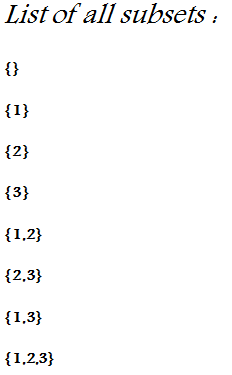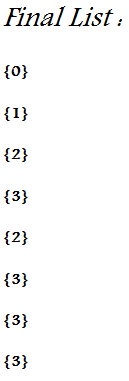Xsquare And Number List
Tag(s):

Binary search algorithm, Implementation, Medium, Sorting algorithm

Problem
Editorial
Analytics

Xsquare loves to play with numbers a lot. Today, he has a multi set S consisting of N integer elements. At first, he has listed all the subsets of his multi set S and later replaced all the subsets with the maximum element present in the respective subset.

For example :

Consider the following multi set consisting of 3 elements S = {1,2,3}.Now, Xsquare wonders that given an integer K how many elements in the final list are greater than ( > ) , less than ( < ) or equals to ( == ) K.

To make this problem a bit more interesting, Xsquare decided to add Q queries to this problem. Each of the queries has one of the following type.

• > K : Count the number of elements X in the final list such that X > K.
• < K : Count the number of elements X in the final list such that X < K.
• = K : Count the number of elements X in the final list such that X == K.

Note:

• Answer to a particular query can be very large. Therefore, Print the required answer modulo 109+7.
• An empty subset is replaced by an integer 0.

Input

First line of input contains two space separated integers N and Q denoting the size of multiset S and number of queries respectively. Next line of input contains N space separated integers denoting elements of multi set S. Next Q lines of input contains Q queries each having one of the mentioned types.

Output

For each query, print the required answer modulo 109+7.

Constraints:

• 1 ≤ N,Q ≤ 5*105
• 1 ≤ K,Si ≤ 109
• query_type = { < , > , = }

Warning :

Prefer to use printf / scanf instead of cin / cout.

SAMPLE INPUT
3 5
1 2 3
< 1
> 1
= 3
= 2
> 3

SAMPLE OUTPUT
1
6
4
2
0
Explanation

Refer to the above list for verification of the sample test cases.

Time Limit: 1.0 sec(s) for each input file.
Memory Limit: 256 MB
Source Limit: 1024 KB
Marking Scheme: Marks are awarded when all the testcases pass.
Allowed Languages: Bash, C, C++, C++14, Clojure, C#, D, Erlang, F#, Go, Groovy, Haskell, Java, Java 8, JavaScript(Rhino), JavaScript(Node.js), Julia, Kotlin, Lisp, Lisp (SBCL), Lua, Objective-C, OCaml, Octave, Pascal, Perl, PHP, Python, Python 3, R(RScript), Racket, Ruby, Rust, Scala, Swift, Swift-4.1, TypeScript, Visual Basic

CODE EDITOR

Initializing Code Editor...

This Problem was Asked inChallenge Name

Awesome April '15

OTHER PROBLEMS OF THIS CHALLENGE
• Data Structures > Hash Tables
• Algorithms > Dynamic Programming
• Data Structures > Advanced Data Structures
• Algorithms > Graphs